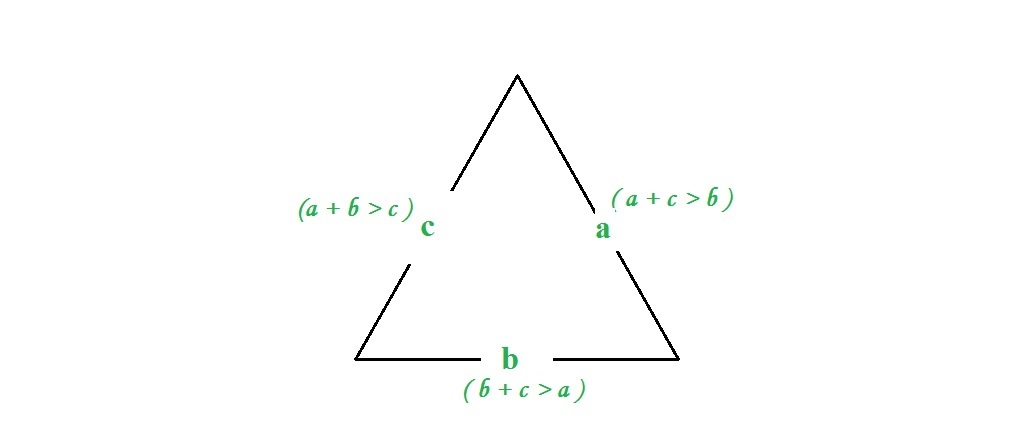# Check whether triangle is valid or not if sides are given

Given three sides, check whether triangle is valid or not.

Examples:

```Input :  a = 7, b = 10, c = 5
Output : Valid

Input : a = 1 b = 10 c = 12
Output : Invalid
```

## Recommended: Please try your approach on {IDE} first, before moving on to the solution.

Approach: A triangle is valid if sum of its two sides is greater than the third side. If three sides are a, b and c, then three conditions should be met.

```1.a + b > c
2.a + c > b
3.b + c > a
```## C++

 `// C++ program to check if three ` `// sides form a triangle or not ` `#include ` `using` `namespace` `std; ` ` `  `// function to check if three sider ` `// form a triangle or not ` `bool` `checkValidity(``int` `a, ``int` `b, ``int` `c) ` `{ ` `    ``// check condition ` `    ``if` `(a + b <= c || a + c <= b || b + c <= a) ` `        ``return` `false``; ` `    ``else` `        ``return` `true``; ` `} ` ` `  `// Driver function ` `int` `main() ` `{ ` `    ``int` `a = 7, b = 10, c = 5; ` `     `  `    ``if` `(checkValidity(a, b, c)) ` `        ``cout << ``"Valid"``; ` `    ``else` `        ``cout << ``"Invalid"``;     ` `} `

## Java

 `// Java program to check  ` `// validity of any triangle ` ` `  `public` `class` `GFG { ` ` `  ` `  `    ``// Function to calculate for validity ` `    ``public` `static` `int` `checkValidity(``int` `a, ` `                                ``int` `b, ``int` `c) ` `    ``{ ` `        ``// check condition ` `        ``if` `(a + b <= c || a + c <= b || b + c <= a) ` `            ``return` `0``; ` `        ``else` `            ``return` `1``; ` `    ``} ` ` `  `    ``// Driver function ` `    ``public` `static` `void` `main(String args[]) ` `    ``{ ` ` `  `        ``int` `a = ``7``, b = ``10``, c = ``5``; ` `     `  `        ``// function calling and print output ` `        ``if` `((checkValidity(a, b, c)) == ``1``) ` `            ``System.out.print(``"Valid"``); ` `        ``else` `            ``System.out.print(``"Invalid"``); ` `         `  `    ``} ` `} ` ` `  `// This article is contributed by 'Akansh Gupta' `

## Python3

 `# Python3 program to check if three ` `# sides form a  triangle or not  ` ` `  `# function to check if three sides  ` `# form a triangle or not  ` `def` `checkValidity(a, b, c):  ` `     `  `    ``# check condition  ` `    ``if` `(a ``+` `b <``=` `c) ``or` `(a ``+` `c <``=` `b) ``or` `(b ``+` `c <``=` `a) : ` `        ``return` `False` `    ``else``: ` `        ``return` `True`         ` `  `# driver code  ` `a ``=` `7` `b ``=` `10` `c ``=` `5` `if` `checkValidity(a, b, c): ` `    ``print``(``"Valid"``)  ` `else``: ` `    ``print``(``"Invalid"``) `

## C#

 `// C# program to check  ` `// validity of any triangle ` `using` `System; ` ` `  `class` `GFG { ` `     `  `    ``// Function to calculate for validity ` `    ``public` `static` `int` `checkValidity(``int` `a, ``int` `b,  ` `                                    ``int` `c) ` `    ``{ ` `         `  `        ``// check condition ` `        ``if` `(a + b <= c || a + c <= b ||  ` `                            ``b + c <= a) ` `            ``return` `0; ` `        ``else` `            ``return` `1; ` `    ``} ` ` `  `    ``// Driver code ` `    ``public` `static` `void` `Main() ` `    ``{ ` `        ``int` `a = 7, b = 10, c = 5; ` `     `  `        ``// function calling and print output ` `        ``if` `((checkValidity(a, b, c)) == 1) ` `          ``Console.Write(``"Valid"``); ` `        ``else` `          ``Console.Write(``"Invalid"``); ` `         `  `    ``} ` `} ` ` `  `// This code is contributed by Nitin Mittal. `

## PHP

 ` `

Output :

```Valid
```

Attention reader! Don’t stop learning now. Get hold of all the important DSA concepts with the DSA Self Paced Course at a student-friendly price and become industry ready.

My Personal Notes arrow_drop_upCheck out this Author's contributed articles.

If you like GeeksforGeeks and would like to contribute, you can also write an article using contribute.geeksforgeeks.org or mail your article to contribute@geeksforgeeks.org. See your article appearing on the GeeksforGeeks main page and help other Geeks.

Please Improve this article if you find anything incorrect by clicking on the "Improve Article" button below.